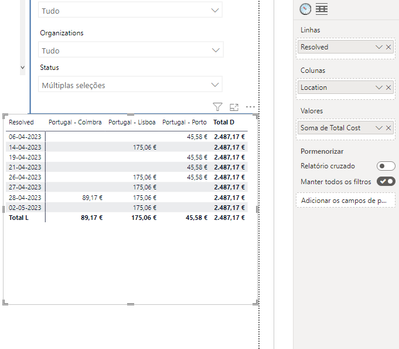cancel
Showing results for
Did you mean:New Member

## Creating matrix from two tables and a calculation

Hello,

I'm trying to do a matrix with 2 tables, but it doesnt do the calculation properly.

This are my 2 tables:Where JIRA.Intervention Area = LocationsCoordinates.Location

On the matrix, the total cost is incorrect (single values and totals):(JIRA.Resolved; LocationsCoordinates.Location; LocationsCoordinates.TotalCost)

Here we can see that, for example, on 14-04-2023 there is 7 rows in "Portugal - Lisboa":The correct calculation for this ex should be 7 * 175,06€.

Also the totals are incorrect too.

1 ACCEPTED SOLUTIONSuper User

There is only one row in 'Locations Coordinates' for an associated TotalCost even as your filter context may have multiple rows from 'JIRA'.

To force PBI to sum 'Locations Coordinates'[TotalCost] for each row of JIRA, you'll need an iterator:

``````Measure =
SUMX(
JIRA,
RELATED( 'Locations Coordinates'[TotalCost] )
)``````

5 REPLIES 5New Member

Thank you for your reply. I will try to explain my case better. In JIRA table I have the date and the location that was made by someone. In the another table (LocationsCoordinate), I have every location with the costs. So, in JIRA will appear the same locatiom multiple times, but in LocationsCoordinate only once with the costs. I'm trying to, for every day that's in JIRA, calculate the costs that's in LocationsCoordinate. (I want the fisrt matrix. The second is just to demonstrate that was 7 dislocation to "Lisboa" for example (COUNT). The correct value should be 7* cost).Super User

If I correctly understand then @MarkLaf 's proposed solution should provide you with the result that you're looking for.Super User

There is only one row in 'Locations Coordinates' for an associated TotalCost even as your filter context may have multiple rows from 'JIRA'.

To force PBI to sum 'Locations Coordinates'[TotalCost] for each row of JIRA, you'll need an iterator:

``````Measure =
SUMX(
JIRA,
RELATED( 'Locations Coordinates'[TotalCost] )
)``````New Member

Thank you. This work for me.Super User

strange that the total cost column is in the LocationCoordinates table which is a dimension table! Are you sure about that? If so then how the ResolvedDate (which is in the JIRA fact table) is related to the total cost? The location is primary key (unique) in the LocationsCoordinates table therefore I guess in this case each location has a single total value so what does mean to split it over months? Also it is not clear what is the difference between the first and the second matrices?